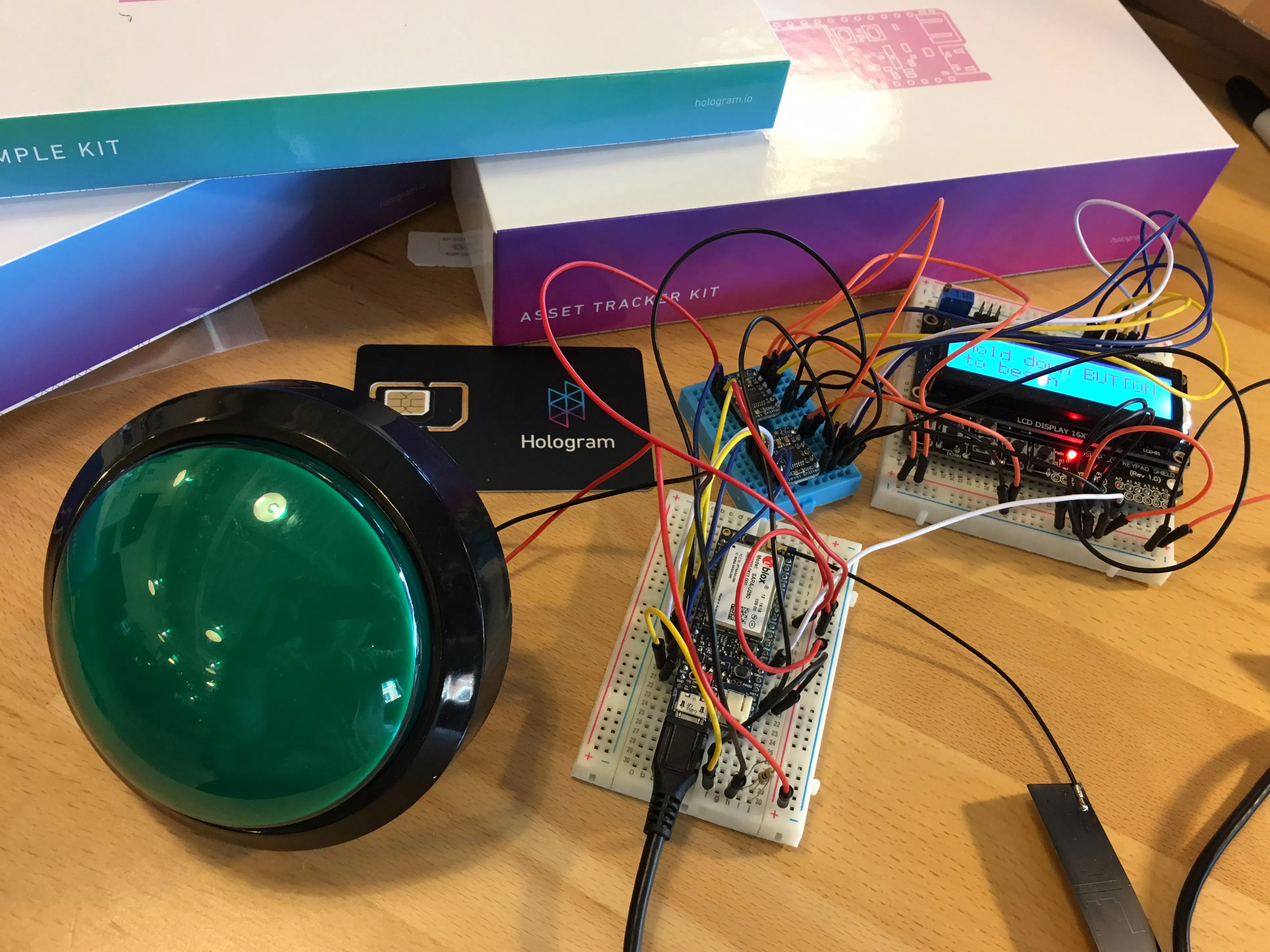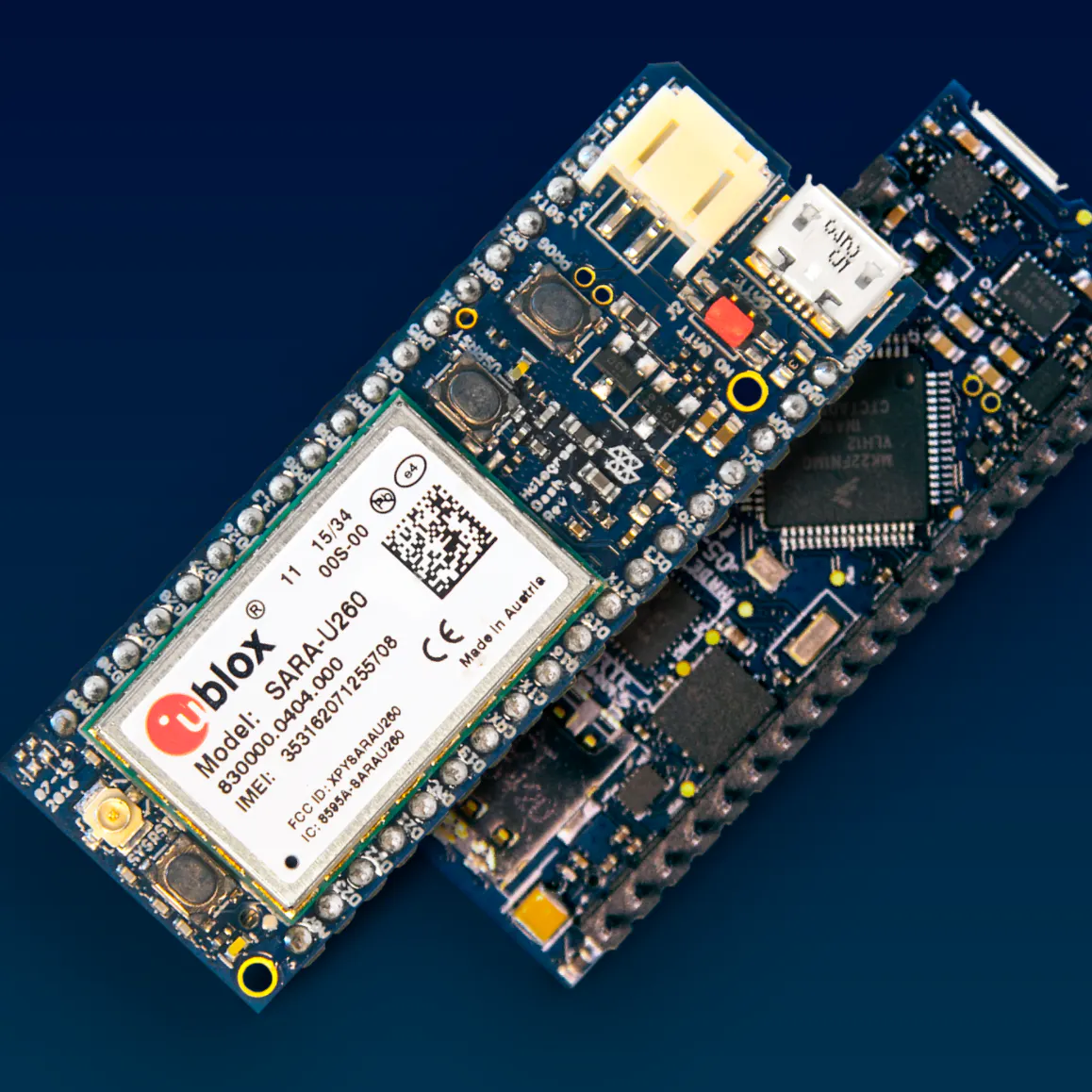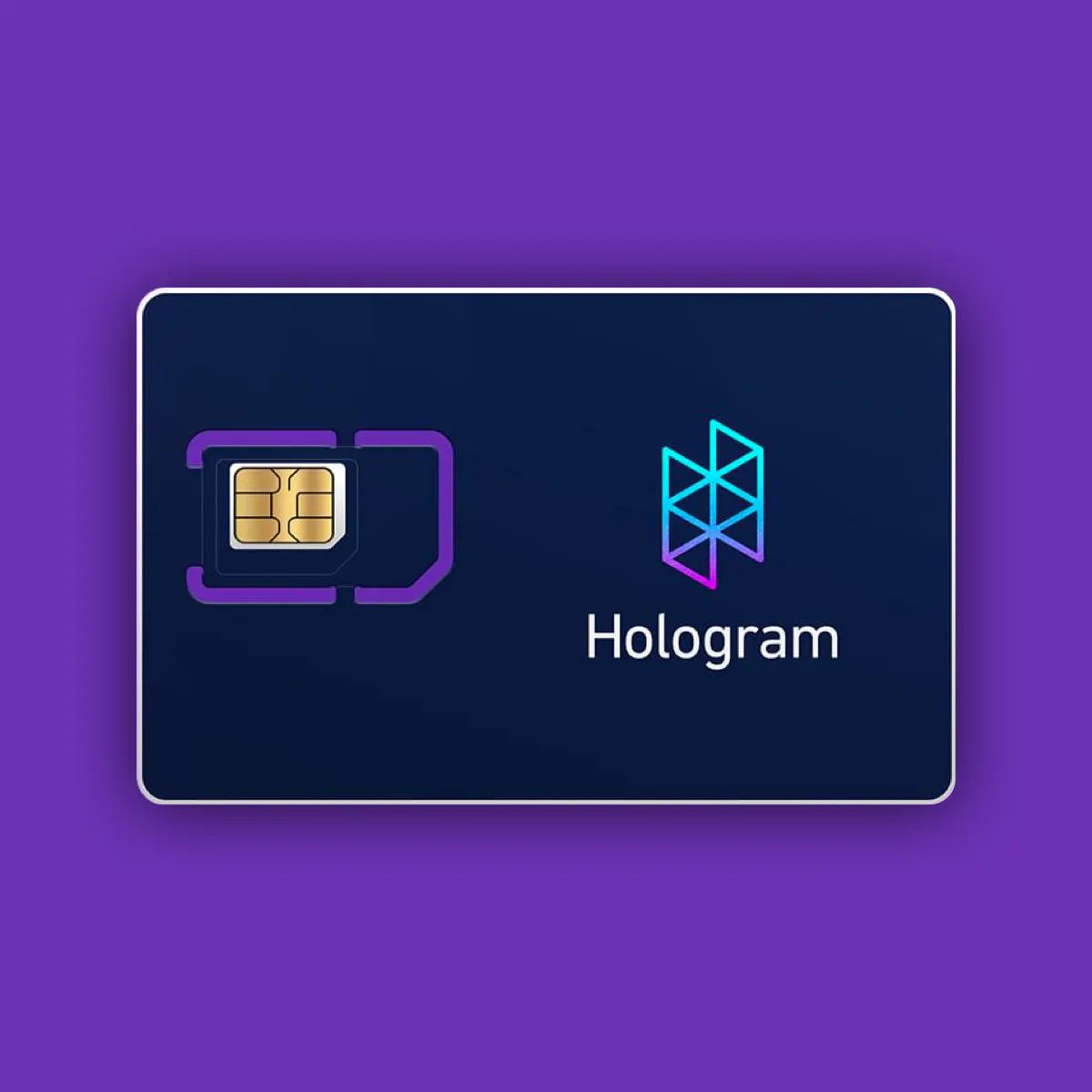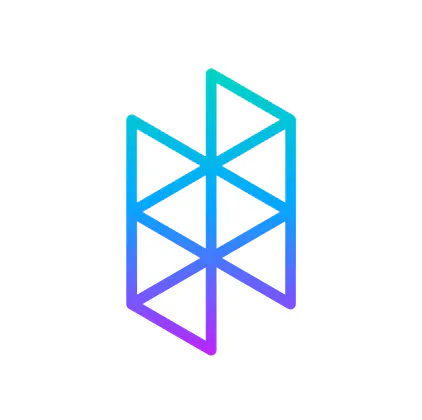Aggro-Crag-in-Real-Life-Button and Leader Board

This is a cellular-connected internet button placed in a remote location. Hit the button, enter your initials, and get recognized.

BeginnerFull instructions provided1 hour2,187Things used in this project

Hardware componentsHologram Dash The Hologram Dash v1.1 is the brains and connectivity for this project
×1Hologram Global IoT SIM Card M2M / IoT SIM that connects this device directly to the Hologram cloud
×1
×1
 Adafruit Bi-Directional Level Shifter
×1Arduino UNO & Genuino UNO Only used for a 5V output
×1
 LCD Display - 16x2 Made by Osepp - works at 5V
×1Resistor 1k ohm
×1Jumper wires (generic)
×25

Software apps and online servicesHologram Data RouterArduino IDE

Hand tools and fabrication machines

 Multimeter Only needed to help you troubleshoot

Code

Dash Sketch on Arduino IDE

Arduino
This is the main sketch for the Aggro-Crag-in-Real-Life-Button
//Sample using LiquidCrystal library
#include <LiquidCrystal.h>

// select the pins used on the LCD panel
//LiquidCrystal lcd(8, 9, 4, 5, 6, 7);  //original library pins
LiquidCrystal lcd(D17, D18, D11, D12, D13, D14);

//set up number of sends to cloud
int numSends = 0; // count number of sends

//set up the big green button
int big_button_in = 0;

//network connection boot sequence variables
int bootSequence = 0;
bool cloudReady = false;
char currChar;
String tempBuffer;
int plusSigns = 0;

// define some values used by the panel and buttons
int lcd_key     = 0;
int adc_key_in  = 0;
#define btnRIGHT  0
#define btnUP     1
#define btnDOWN   2
#define btnLEFT   3
#define btnSELECT 4
#define btnNONE   5

// read the buttons
{
adc_key_in = analogRead(A0);      // read the value from the sensor
// my buttons when read are centered at these valies: 0, 144, 329, 504, 741
// we add approx 50 to those values and check to see if we are close
if (adc_key_in > 1000) return btnNONE; // We make this the 1st option for speed reasons since it will be the most likely result
if (adc_key_in < 60)   return btnRIGHT;
if (adc_key_in < 200)  return btnUP;
if (adc_key_in < 450)  return btnDOWN;
if (adc_key_in < 640)  return btnLEFT;
if (adc_key_in < 970)  return btnSELECT;
return btnNONE;  // when all others fail, return this...
}

//define alphabet array
char alphaArray = {'A', 'B', 'C', 'D', 'E', 'F', 'G', 'H', 'I', 'J', 'K', 'L', 'M', 'N', 'O', 'P', 'Q', 'R', 'S', 'T', 'U', 'V', 'W', 'X', 'Y', 'Z'};
int currentAlphaPosition = 0;

int currentCursorPosition = 0;
char initials = {'A','A','A'};

int cursorTracker = {0,0,0};
int sentToCloudFlag = 0;

int nextAlphaPosition(int current, int lcd_key){
int next = current;

if(lcd_key == btnUP){
next = current + 1;
}
else if(lcd_key == btnDOWN){
next = current - 1;
}

if (next == 26){
next = 0;
}

if (next == -1){
next = 25;
}

return next;

}

int nextCursorPosition(int current, int lcd_key){
int next = current;

if(lcd_key == btnRIGHT){
next = current + 1;
}
else if(lcd_key == btnLEFT){
next = current - 1;
}

if (next == 3){
next = 0;
}

if (next == -1){
next = 2;
}

return next;

}

//debounce setup
// Variables will change:
int buttonState;             // the current reading from the input button
int lastButtonState = btnNONE;   // the previous reading from the input pin

// the following variables are long's because the time, measured in miliseconds,
// will quickly become a bigger number than can be stored in an int.
long lastDebounceTime = 0;  // the last time the output pin was toggled
long debounceDelay = 50;    // the debounce time; increase if the output flickers

//lcd state machine flags
int scene1 = 0;
int scene2 = 0;
int scene3 = 0;
int scene4 = 0;
int scene5 = 0;

void setup()
{
lcd.begin(16, 2);              // start the library
lcd.setCursor(0,0);
lcd.print("Booting..."); // print a simple message

Serial.begin(9600);
SerialCloud.begin(115200);
}

void loop()
{

//lcd.setCursor(9,1);            // move cursor to second line "1" and 9 spaces over
//lcd.print(millis()/1000);      // display seconds elapsed since power-up

//connection boot
if(bootSequence == 0){
char currChar;

while (SerialCloud.available()) {

if (currChar == '+') {
plusSigns++;
} else {
plusSigns = 0;
}

if (plusSigns == 3) {
scene1=1;
bootSequence=1;
lcd.clear();
}

SerialUSB.write(currChar);
Serial2.write(currChar);
}
}

if (scene1 == 1){
delay(1000);
Serial.println("Entering Scene 1");
lcd.setCursor(0,0);
lcd.print("Hold down BUTTON");

lcd.setCursor(0,1);
lcd.print("to begin");

if (big_button_in >  500){
scene1 = 0;
scene2 = 1;
lcd.clear();
}
}

if (scene2 == 1){
lcd.setCursor(0,0);
lcd.print("Congrats! Press");

lcd.setCursor(0,1);
lcd.print("SELECT");

//just recopy a bunch of code because refactoring is hard...
lcd_key = read_LCD_buttons();  // read the buttons

// If the switch changed, due to noise or pressing:
if (lcd_key != lastButtonState) {
// reset the debouncing timer
lastDebounceTime = millis();
}

if ((millis() - lastDebounceTime) > debounceDelay) {

// if the button state has changed:
if (lcd_key != buttonState) {
buttonState = lcd_key;
if (lcd_key == btnSELECT){
scene2 = 0;
scene3 = 1;
lcd.clear();
lcd.setCursor(0,0);
Serial.println("Select pressed");
}
}
}

// it'll be the lastButtonState:
lastButtonState = lcd_key;
}

if (scene3 == 1){
lcd.setCursor(0,1);            // move to the begining of the second line
lcd_key = read_LCD_buttons();  // read the buttons

lcd.setCursor(0,0);
lcd.print("Enter initials");

// If the switch changed, due to noise or pressing:
if (lcd_key != lastButtonState) {
// reset the debouncing timer
lastDebounceTime = millis();
}

if ((millis() - lastDebounceTime) > debounceDelay) {

// if the button state has changed:
if (lcd_key != buttonState) {

//Serial.println("button state has changed");
buttonState = lcd_key;

currentCursorPosition = nextCursorPosition(currentCursorPosition, lcd_key);
currentAlphaPosition = nextAlphaPosition(cursorTracker[currentCursorPosition], lcd_key);
cursorTracker[currentCursorPosition] = currentAlphaPosition;

initials[currentCursorPosition] = alphaArray[currentAlphaPosition];
Serial.print(initials);
Serial.print(initials);
Serial.println(initials);

if (lcd_key == btnRIGHT){
Serial.print("Right");
Serial.println(currentCursorPosition);
}
else if (lcd_key == btnUP){
Serial.print("Up");
Serial.println(alphaArray[currentAlphaPosition]);
}
else if (lcd_key == btnDOWN){
Serial.print("Down");
Serial.println(alphaArray[currentAlphaPosition]);
}
else if (lcd_key == btnLEFT){
Serial.print("Left");
Serial.println(currentCursorPosition);
}
else if (lcd_key == btnSELECT){
Serial.print("Select");
scene3 = 0;
scene4 = 1;
}
}

}

// it'll be the lastButtonState:
lastButtonState = lcd_key;

lcd.setCursor(0,1);
lcd.print(initials);
lcd.setCursor(1,1);
lcd.print(initials);
lcd.setCursor(2,1);
lcd.println(initials);
}

if (scene4 == 1){
lcd.setCursor(0,0);
lcd.print("Sending. Go to");

lcd.setCursor(0,1);
lcd.print("hologram.io");
String cloudInitials = initials;

// Try to send data 3 times to cloud
if(numSends < 3) {
SerialUSB.println("Sending a message to the Cloud...");
SerialCloud.println(cloudInitials);
SerialUSB.println(cloudInitials);
SerialUSB.println("Message sent!");
numSends++; // increase the number-of-sends counter
}

if(numSends >= 3){
scene4 = 0;
scene5 = 1;
}

// it'll be the lastButtonState:
lastButtonState = lcd_key;
}

if (scene5 == 1){
lcd.clear();
lcd.setCursor(0,0);
lcd.print("Sending. Go to");

lcd.setCursor(0,1);
lcd.print("hologram.io");

delay(7500);

lcd.clear();
lcd.setCursor(0,0);
lcd.print("Resetting...");

lcd.setCursor(0,1);
lcd.print("Goodbye!");
delay(5000);
lcd.clear();
scene5 = 0;
scene1 = 1;

//Reset variables
currentAlphaPosition = 0;

currentCursorPosition = 0;
initials='A';
initials='A';
initials='A';

cursorTracker=0;
cursorTracker=0;
cursorTracker=0;
sentToCloudFlag = 0;
numSends = 0;

}

}

Credits

Derrick Wolbert

1 project • 1 follower

Sheena Martin

1 project • 0 followers
Content Coordinator for Hologram.io

benstr 🚀

4 projects • 85 followers
Developer Advocate @ Hologram.io - We make cellular IoT easy.